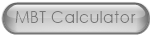The Cement Grinding Office
The Art Of Sharing and...Imagination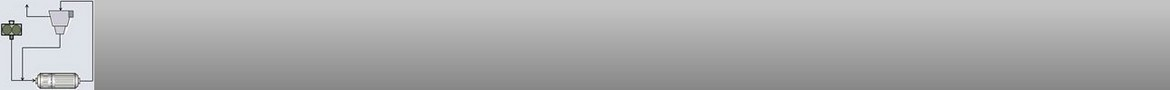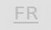- Ball charges: This calculator gives the surface and the average weight of the ball charges. It gives also a rough interpretation of the ball charge efficiency:
- Ball top size (bond formula): calculation of the top size grinding media (balls or cylpebs):
- Modification of the Ball Charge: This calculator analyses the granulometry of the material inside the mill and proposes a modification of the ball charge in order to improve the mill efficiency:
- Ball charges composition: These calculators give the gradation of the ball charge in function of predefined conditions:
- Ball charge make-up: This calculator gives the percentage for each diameter of balls when there is a make-up schedule: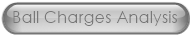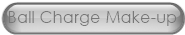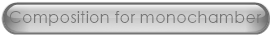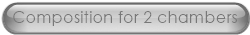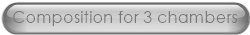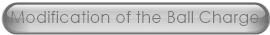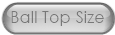- Marked Ball Test Calculator: Two tables to collect the data of a marked ball test with calculation of the wear rates: